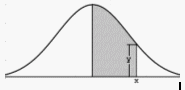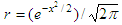# Online Normal Distribution Calculator

This calculator for Normal distribution asks to enter three parameters: the known mean of your distribution, its standard deviation and the value you want to explore. In return, the outputs will be the frequency and the probability of the value under study.Normal Distribution

The shaded area represents the probability of "x’" and its frequency is "y".
The normal probability is approximated using the following formula:

probability = 1 – r(a1t + a2t2 + a3t3) + eps

where:
a1 = 0.4361836
a2 = -0.1201676
a3 = 0.9372980t = 1/(1 + 0.33267x)

eps < 10-5

Example:

The average frequency of a certain type of computers in manufacturing is 2 GHz. The known standard deviation is 0.02 GHz. If the frequency of those computers is normally distributed, what is the probability that a computer runs between 2 GHz and 2.01 GHz?

Run the calculator below and enter...

Enter mean: 2
Enter std. deviation: 0.02
Enter variable: 2.01

The output is

Frequency =     0.3521
Probability =     0.6915